# Simple code generation

This tutorial aims at presenting the new Scilab Code Generator:

https://atoms.scilab.org/toolboxes/xcos_code_generator/

More details on:

https://www.scilab.org/software/atoms/scilab-code-generator

## Simple example: Gaussian filter

A gaussian filter s a filter whose impulse response is a Gaussian function (Wikipedia)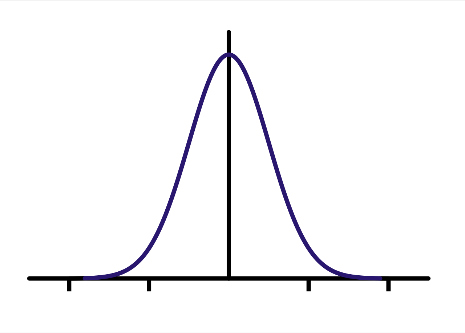Let us write a simple script describing the call of a gaussian filter, on a matrix B of size 10x10.

`function B=gauss_filter_3_3(A)`
`   x=size(A,2);`
`   y=size(A,1);`
`   B = zeros(y, x);`
`   for j = 2:y-1`
`       for i= 2:x-1`
`           val= 4*A(j,i)+2*(A(j,i-1)+A(j,i+1)+A(j+1,i)+A(j-1,i))+A(j+1,i+1)+A(j-1,i+1)+A(j+1,i-1)+A(j-1,i-1);`
`           B(j,i) = val/16;`
`       end`
`   end`
`endfunction`
` `
`A = rand(10, 10) * 256;`
```B = gauss_filter_3_3(A);

```

If you represent A & B with the function Matplot, you will clearly understand the impact of the Gaussian filter function: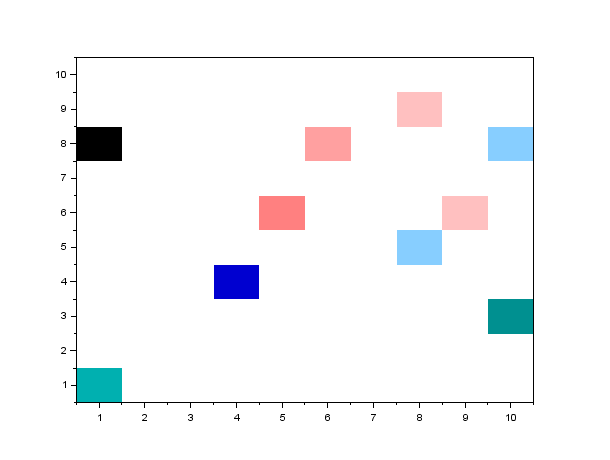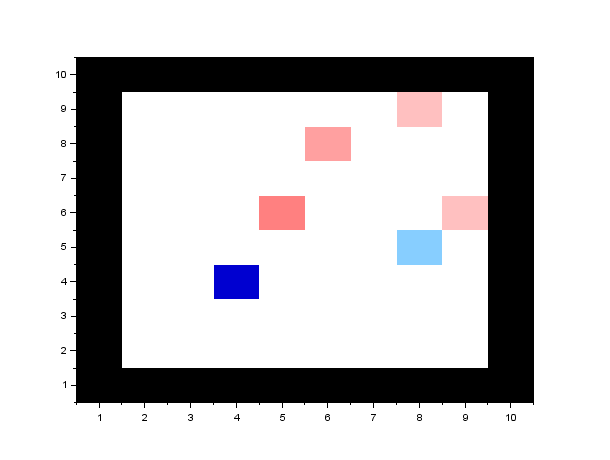(A is on the left, and B on the right)

Also, you can have a look at the data in the variable editor: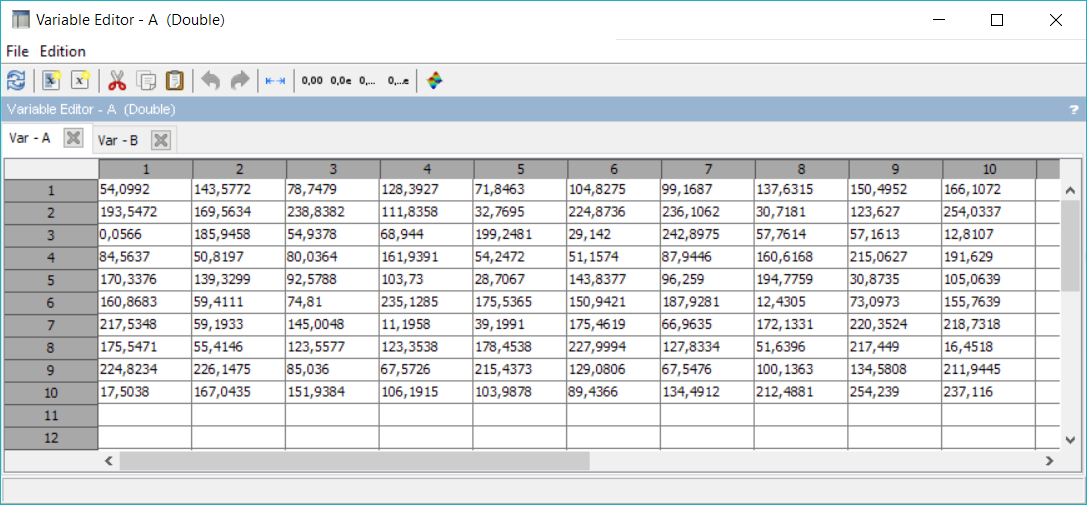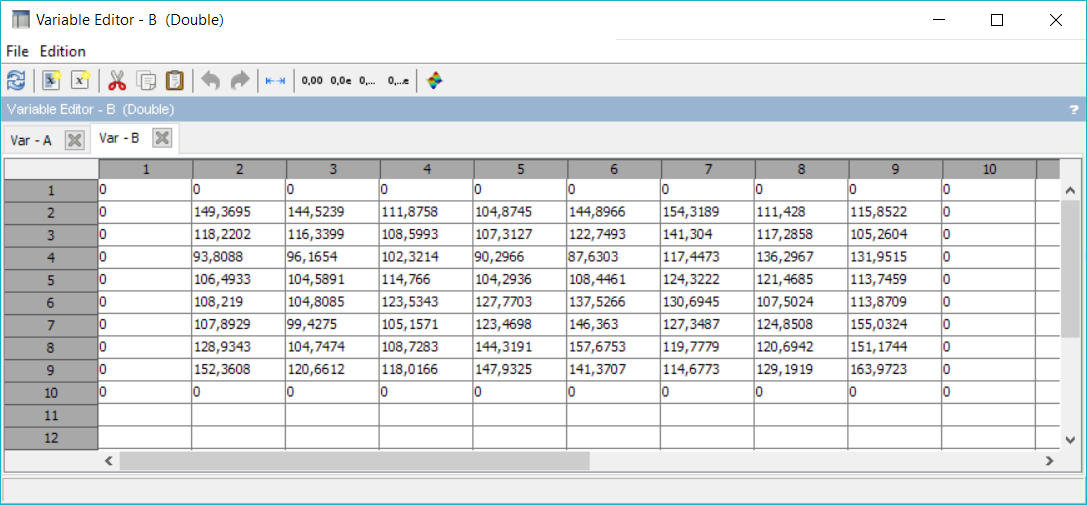First important thing to notice is that you need to clarify the calling sequences of the code you aim at generating. You cannot simply generate a function (or a .sci file). The code generator needs to know here that the gauss_filter function will be called by a matrix of double with a size 10x10.

Once the calling sequences is clarified, you can generate the code:

```--> emx_codegen('gauss_filter.sce')

emmtrix Code Generator (Oct 26 2017 01,14,08)

gauss_filter.sce(14,1-2), W00053 Warning, Variable B not used.

B = gauss_filter_3_3(A);

~

Value Range Coverage, 147/147

Memory Stats, 8 stack, 0 heap, 0 global

Compile time, 160 ms

Build succeeded ... 0 error(s), 1 warning(s), 0 note(s)

Retrieving file Makefile.gen

Retrieving file emx_codegen.h

Retrieving file emx_codegen_intern.h

Retrieving file emx_codegen_rand.c

Retrieving file emx_codegen_rand.h

Retrieving file gauss_filter.c

Retrieving file gauss_filter.c.map

Retrieving file gauss_filter.d

Retrieving file gauss_filter.h

Retrieving file gauss_filter.h.map

Retrieving file gauss_filter.html

Retrieving file gauss_filter.sce

ans  =

[]```

The code generator is quite chatty. You can see that many results are returned in a folder called build by default. This is how the generated code looks like: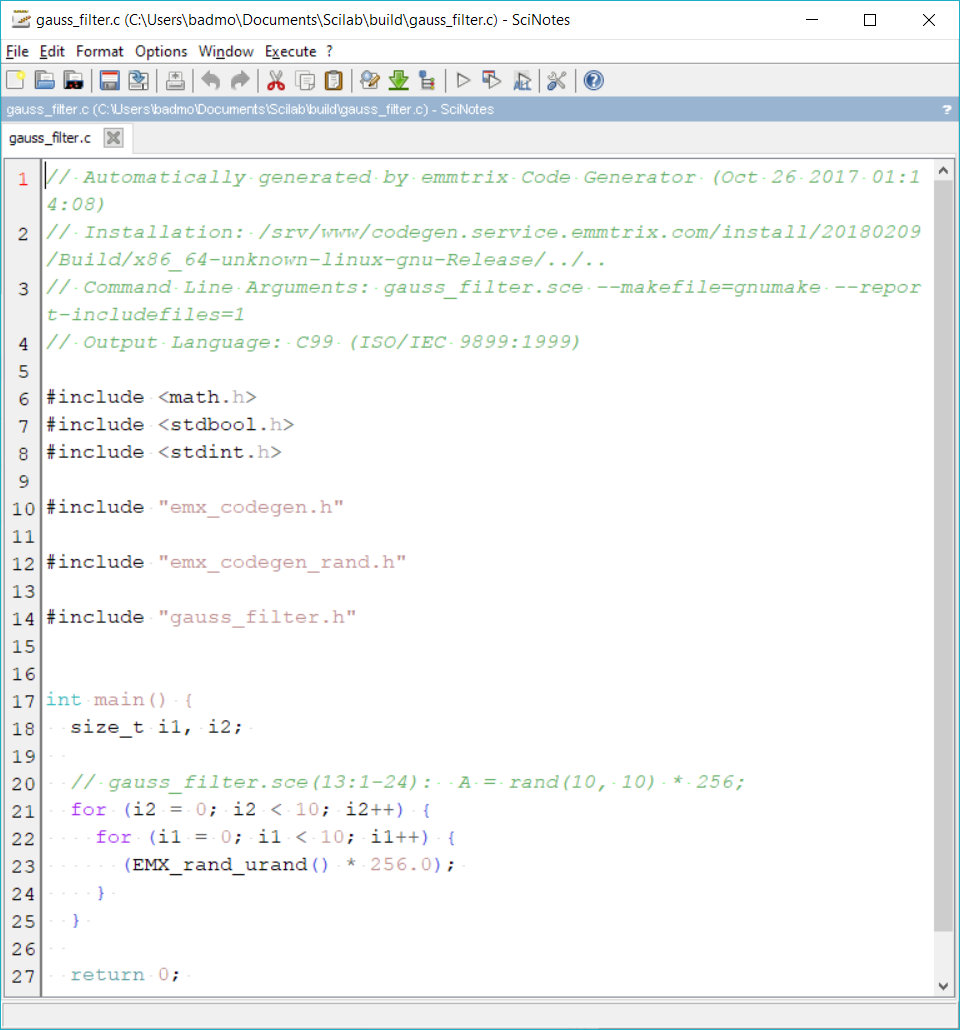## Display the output

If you now simply add a call to the variable B at the last line of your Scilab script and regenerate the code, you will end up with this:

`// Automatically generated by emmtrix Code Generator (Oct 26 2017 01:14:08)`
`// Installation: /srv/www/codegen.service.emmtrix.com/install/20180209/Build/x86_64-unknown-linux-gnu-Release/../..`
`// Command Line Arguments: gauss_filter.sce --makefile=gnumake --report-includefiles=1`
`// Output Language: C99 (ISO/IEC 9899:1999)`
```
```
`#include `
`#include `
`#include `
`#include `
```
```
`#include "emx_codegen.h"`
```
```
`#include "emx_codegen_rand.h"`
```
```
`#include "gauss_filter.h"`
```
```
`void gauss_filter_3_3(double B_data, double A_data) {`
`  size_t i1, i2, i3, i4; `
`  double val_data; `
`  `
`  // gauss_filter.sce(4:4-20):  B = zeros(y, x);`
`  #pragma EMX_KILLVAR B_data`
`  `
`  for (i2 = 0; i2 < 10; i2++) {`
`    for (i1 = 0; i1 < 10; i1++) {`
`      B_data[i2][i1] = 0; `
`    } `
`  } `
`  `
`  // gauss_filter.sce(5-10):  for j = 2:y-1`
`  `
`  for (i3 = 0; i3 < 8; i3++) {`
`    // gauss_filter.sce(6-9):  for i= 2:x-1`
`    `
`    for (i4 = 0; i4 < 8; i4++) {`
`      // gauss_filter.sce(7:12-110):  val= 4*A(j,i)+2*(A(j,i-1)+A(j,i+1)+A(j+1,i)+A(j-1,i))+A(j+1,i+1)+A(j-1,i+1)+A(j+1,i-1)+A(j-1,i-1);`
`      `
`      val_data = 4.0 * A_data[(int32_t )(i4) + 1][(int32_t )(i3) + 1] + 2.0 * (A_data[(int32_t )(i4)][(int32_t )(i3) + 1] + A_data[(int32_t )(i4) + 2][(int32_t )(i3) + 1] + A_data[(int32_t )(i4) + 1][(int32_t )(i3) + 2] + A_data[(int32_t )(i4) + 1][(int32_t )(i3)]) + A_data[(int32_t )(i4) + 2][(int32_t )(i3) + 2] + A_data[(int32_t )(i4) + 2][(int32_t )(i3)] + A_data[(int32_t )(i4)][(int32_t )(i3) + 2] + A_data[(int32_t )(i4)][(int32_t )(i3)]; `
`      `
`      // gauss_filter.sce(8:12-28):  B(j,i) = val/16;`
`      B_data[(int32_t )(i4) + 1][(int32_t )(i3) + 1] = val_data / 16.0; `
`    } `
`  } `
`} `
```
```
`int main() {`
`  static double A_data, B_data; `
`  size_t i1, i2, i3, i4; `
`  `
`  // gauss_filter.sce(13:1-24):  A = rand(10, 10) * 256;`
`  #pragma EMX_KILLVAR A_data`
`  `
`  for (i2 = 0; i2 < 10; i2++) {`
`    for (i1 = 0; i1 < 10; i1++) {`
`      A_data[i2][i1] = EMX_rand_urand() * 256.0; `
`    } `
`  } `
`  `
`  // gauss_filter.sce(14:1-25):  B = gauss_filter_3_3(A);`
`  gauss_filter_3_3(B_data, A_data); `
`  `
`  // gauss_filter.sce(15:1-2):  B`
`  puts("B = "); `
`  for (i3 = 0; i3 < 10; i3++) {`
`    for (i4 = 0; i4 < 10; i4++) {`
`      printf(" %11.6g", B_data[i4][i3]); `
`    } `
`    puts(""); `
`  } `
`  `
`  return 0; `
`}`

You can compile this C code, and verify that it is working well:

(Pay attention: in this example, the random function as been generated as well, and needs to be attached to the program).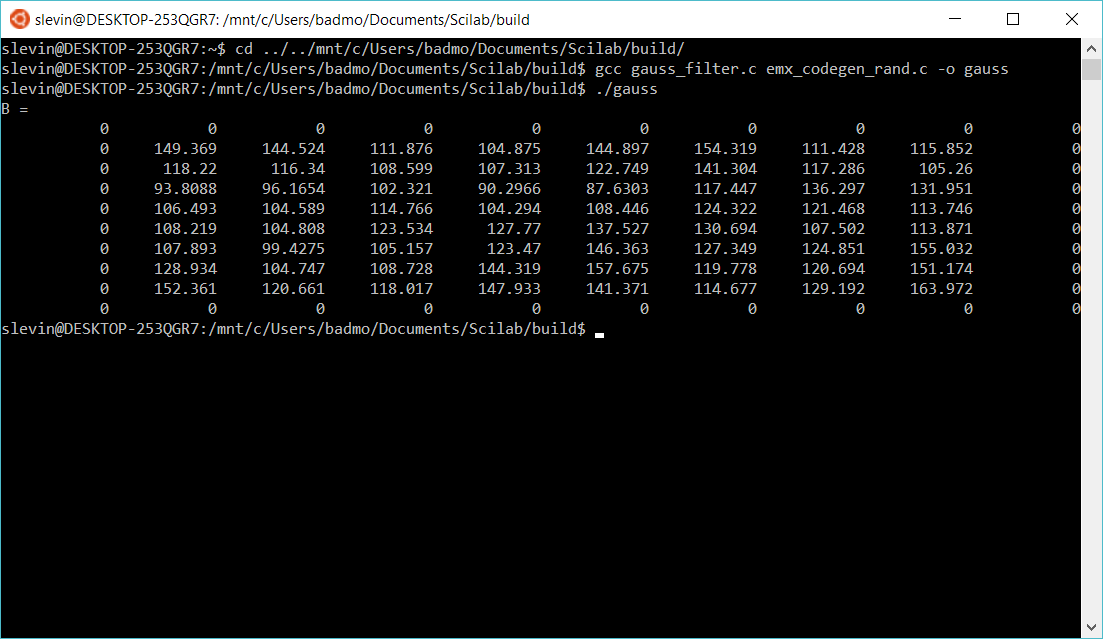You can also create a windows (64bits) executable with this command:

` x86_64-w64-mingw32-gcc -o gauss.exe gauss_filter.c emx_codegen_rand.c`